## HelicopterSciML.jl

Helicopter Scientific Machine Learning (SciML) Challenge Problem
Author SciML
Popularity
32 Stars
Updated Last
2 Years Ago
Started In
July 2020

# Helicopter Scientific Machine Learning (SciML) Challenge ProblemThis is a repository for the helicopter SciML challenge problem. The problem is centered on automatically discovering physically-realistic augmentations to a model of a laboratory helicopter to better predict the movement. This problem is hard because it is realistic:

• We do not have data from every single detail about the helicopter. We know the electrical signals that are being sent to the rotories and we know the measurements of yaw and pitch angles, but there are many hidden variables that are not able to be measured.
• While it is governed by physical first principles, these first principles do not describe the whole system.
• Since our goal is to understand the helicopter system, simply training a neural network or performing reinforcement learning does not solve the problem: we wish to understand the actual physics instead of simply making predictions.

This challenge is an open-ended problem to find realistic equations. The goal of this challenge is to utilize automated tools to discover a physically-explainable model that accurately predicts the dynamics of the system.

## Introduction to the Model, Data, and Challenge

A first principles model for the helicopter systems is derived in the challenge problem write-up. On this system the electrical inputs to the rotories (u(t)) are known and are used to turn up and down the propellers. These then effect the state variables of the system (x(t)), of which the pitch and yaw angles are measured.

The challenge is to predict the future state of the yaw and pitch angles given the current known states and inputs. The challenge is open ended, as in, the best predictor is not necessarily the most useful predictor so a simple number is cannot be used to judge how good a solution is. A neural network can remember and predict the data exactly, but that's not interesting! What we are looking for is new physical equations, augmentations and changes to the original model, that make better predictions and explain the effects (and their importance) which are left out of the original derivation. These augmentations should be physically justifiable (though subjective, rigorous first principles physics should be used to justify the possible explanation for any predictive terms) and should be automatically generated using some programmatic approach. The goal is to figure out how to have computers automatically improve physical equations in a way that can lead to greater understanding of systems from data.

## Initial Results

The first principle physics model makes fairly good predictions for the evolution of the pitch angle but does is not a great predictor of the yaw angle: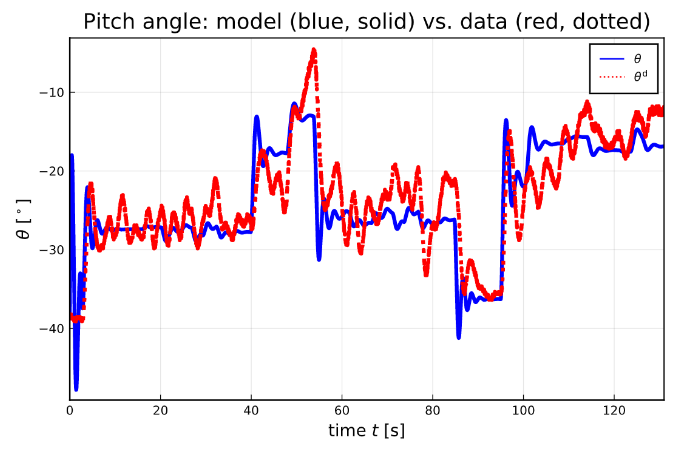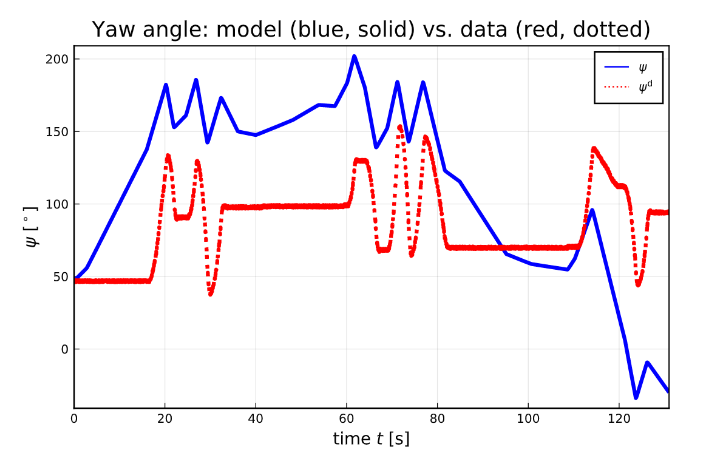Initial attempts at automated discovery at the missing physical equations utilized universal differential equations to discover missing friction terms in ths torque: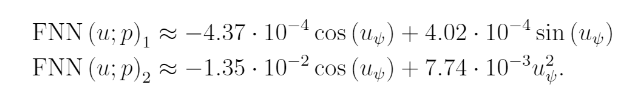The model with this automatically discovered terms has an improved fit to the yaw angle: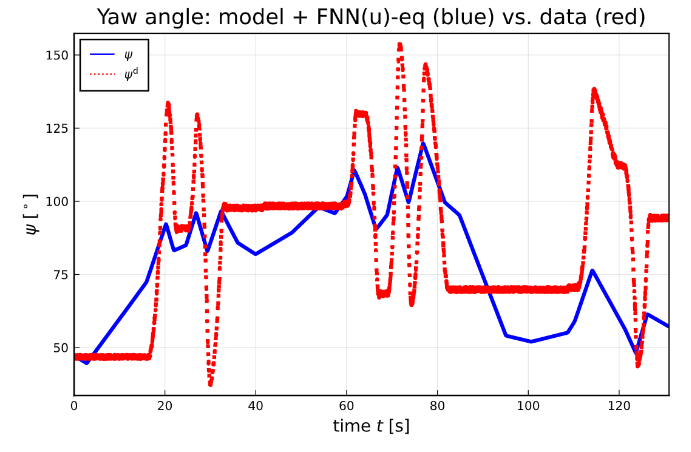Still, it is clear that there are many aspects of the model that can be improved, such as adding deadband effects. A more detailed introduction to the current results can be found in the challenge problem write-up.

## Video Introduction to the Dataset

For an introduction to the dataset, how it was collected, the associated challenges, please see the following video: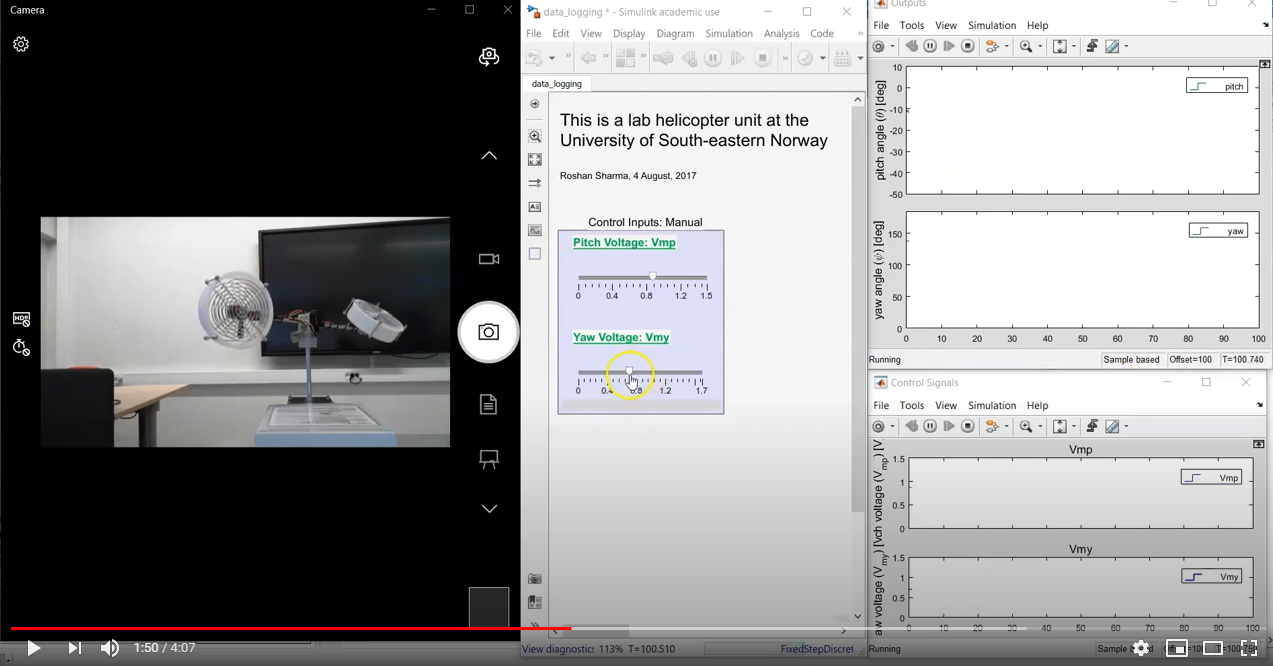## Goals of the Challenge

The challenge is multi-faceted and there is no single number to determine whether one has done well. However, the features of a solution which are beneficial are:

• Predictive: Indicators of fit are given by the predicted pitch and yaw angles.
• Conservative: the new model is closely based in kind or structure to the original mechanistic model. If a very small changes causes a very large benefit, this is seen as advantageous to a change that throws out the mechanism entirely but receives good predictions.
• Physical: the new model should be able to mechanistically justify the terms that are added. Unphysical terms are deemed not desirable even if they add to the predictiveness of the model.
• Extrapolatory: Models trained on a subset of the time that can extrapolate to future times are deemed advantageous to models that utilize the full data.
• Validatable: Models that generate hypotheses that can be independently validated are deemed advantageous to models that are a blackbox and can only be validated by the exact time series data that is used for training/testing.

## Detailed Description of the Challenge Problem

A detailed description of the challenge problem can be found in the challenge problem write-up which explains the derivation of the helicpter model, the data source, the current fits, and the current experiments in automated physical augmentation discovery.

## Starter Code

The scripts, stored in `/scripts`, are as follows:

• Helicopter.jl: the initial global optimization performed using the basic physical equations.
• neural_attempts.jl: the attempted neural augmentation strategies. In `_u` the approach is making K nonlinear in u(t), and `_ux` allows for the addition of new state-dependent terms. Additionally, fourier_attempt showcases using the Fourier basis for learning a similar universal approximator.
• equation_discovery.jl: the sparsification of the discovered neural neural network. Results in the determination of new physical equations and a plot of the accuracy. `_u` is for a version that only is trained to add terms based on u(t), while `_ux` allows state-dependent terms to be discovered.

The other folders are:

• data: the original data
• figs: the figures generated by the scripts
• optimization_results: the values generated by the scripts, like trained neural network parameters and discovered equations
• papers: the work in progress draft

At the top level there is a Project.toml and Manifest.toml for reproducibility.